# Inadequate proof of Bloch's theorem?

• I
Happiness
Suppose a wave function is a linear combination of 2 stationary states: ##\psi(x)=c_1\psi_1(x)+c_2\psi_2(x)##.

By [5.52] and [5.53], we have ##\psi(x+a)=e^{iK_1a}c_1\psi_1(x)+e^{iK_2a}c_2\psi_2(x)##. But to prove [5.49], we need ##K_1=K_2##. That means all the eigenvalues of the "displacement" operator D have to be the same. But why is it so?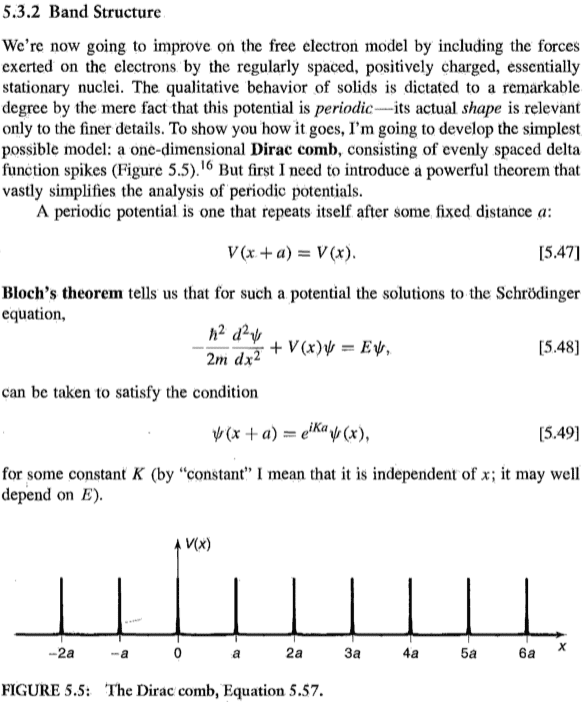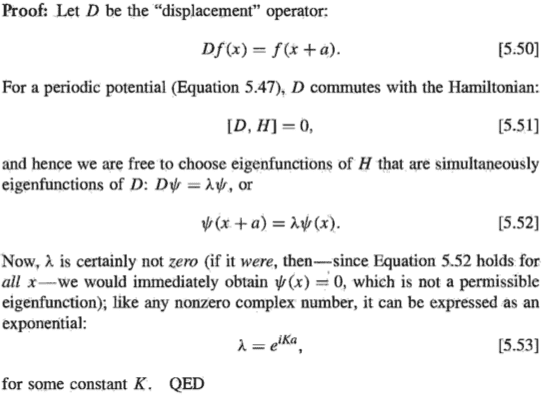Reference: Intro to QM, David J Griffiths, p224

But to prove [5.49], we need ##K_1=K_2##.

That is obviously incorrect. If you do not immediately see why, just plot both parts of the complex exponential function for several K, e.g. K=k, 2k and so on.

•vanhees71
Happiness
That is obviously incorrect. If you do not immediately see why, just plot both parts of the complex exponential function for several K, e.g. K=k, 2k and so on.
I don't understand you. What's the meaning of your k?

We need ##K_1=K_2## (mod ##\frac{2\pi}{a})##.

Last edited:
•vanhees71
Happiness
##K_1=-K_2## (mod ##\frac{2\pi}{a}##) according to another book, QM 2nd ed., Bransden & Joachain, p183, (see (4.196) below).

That would mean that ##\psi(x+a)=D\psi(x)=D[c_1\psi_1(x)+c_2\psi_2(x)]=e^{iK_1a}c_1\psi_1(x)+e^{-iK_1a}c_2\psi_2(x)##. But this contradicts (4.198) of the same book itself.

Note that here ##\psi_1(x)## and ##\psi_2(x)## have the same energy, but could have different ##\lambda##, the eigenvalues for D.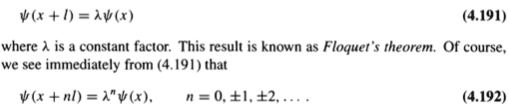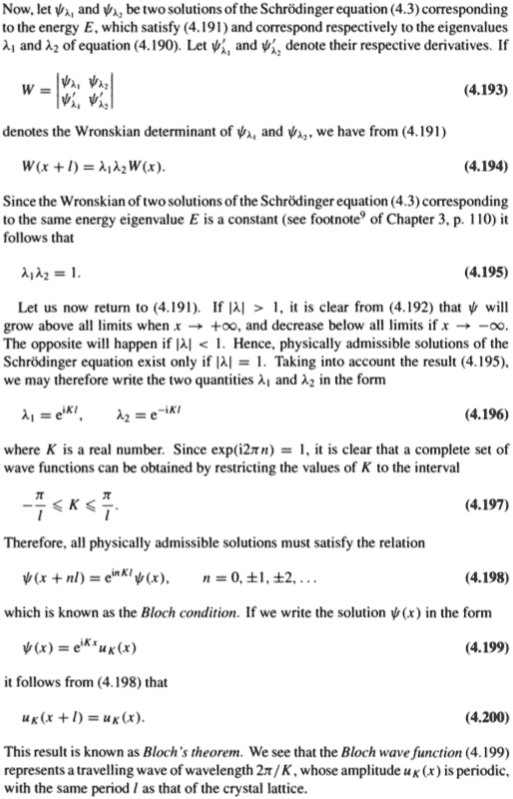Last edited:
I don't understand you. What's the meaning of your k?

Just any wavenumber. Feel free to plot it for some arbitrary number, twice the number and so on.

##K_1=-K_2## according to another book, QM 2nd ed., Bransden & Joachain, p183, (see (4.196) below).

This is not even close to what the book says. Note the restricted range of values chosen in 4.197 and especially the comment between 4.196 and 4.197. Is it even possible that in 5.49 just a single K exists that solves the equation, if exp(2 pi i n)=1?

Happiness
Just any wavenumber. Feel free to plot it for some arbitrary number, twice the number and so on.

This is not even close to what the book says. Note the restricted range of values chosen in 4.197 and especially the comment between 4.196 and 4.197. Is it even possible that in 5.49 just a single K exists that solves the equation, if exp(2 pi i n)=1?

The point about writing explicitly (mod ##\frac{2\pi}{a}##)? Is that all you wanted to say?

(mod ##\frac{2\pi}{a}##) doesn’t resolve the issue.

The point about writing explicitly (mod ##\frac{2\pi}{a}##)? Is that all you wanted to say?

(mod ##\frac{2\pi}{a}##) doesn’t resolve the issue.

It gives you an infinite number of eigenvalues (or rather: equivalent formulations) instead of just a single one. If that does not solve your problem , you should state your problem clearly. The proof in Griffith's book is pretty clear and straightforward.

Happiness
It gives you an infinite number of eigenvalues (or rather: equivalent formulations) instead of just a single one. If that does not solve your problem , you should state your problem clearly. The proof in Griffith's book is pretty clear and straightforward.
It’s possible for two linearly independent common eigenfunctions of the Hamiltonian and D that have the same energy to have different eigenvalues for D, meaning two different λ. But Griffiths assumes they must be the same (modularly). In fact, Bransden and Joachain show that they cannot be the same (modularly), proving Griffiths wrong (unless K=0, the trivial case).

It may be important to understand the implication of the bolden two above. There are exactly two linearly independent eigenfunctions of the same energy, since the Schrondinger equation is second order. And this allows the use of the Wronskian determinant of the 2X2 matrix in B&J's (4.193).

Last edited:
It’s possible for two linearly independent eigenfunctions of the Hamiltonian that have the same energy to have different eigenvalues for D, meaning two different ##\lambda##. But Griffiths assumes they must be the same (modulo-ly). In fact, Bransden and Joachain show that they cannot be the same (modulo-ly), proving Griffiths wrong (unless K=0, the trivial case).

Sorry, but what you claim is far from correct. It starts with your assumption that for a wave function $\psi(x)=c_1\psi_1(x)+c_2\psi_2(x)$, you will get something like $\psi(x+a)=e^{iK_1a}c_1\psi_1(x)+e^{iK_2a}c_2\psi_2(x)$. Of course you would get something similar to $\psi(x+a)=e^{iKa}(c_1\psi_1(x)+c_2\psi_2(x))$. Of course you could create a displacement on two individual functions already displaced to get something like: $\psi(x+a)=e^{iKa}(e^{iK_1a}c_1\psi_1(x)+e^{iK_2a}c_2\psi_2(x))$.

Any K that fulfills the periodicity condition in the proof by Griffith is a solution and of course this includes both signs. He never claims that all eigenvalues have to be the same.

Happiness
Of course you would get something similar to $\psi(x+a)=e^{iKa}(c_1\psi_1(x)+c_2\psi_2(x))$.
Why? The ##K## for ##\psi_1(x)## and ##\psi_2(x)## are different (modularly).
He never claims that all eigenvalues have to be the same.
If the eigenvalues are not the same, you cannot factorise out ##e^{iKa}##!

Last edited:
Why? The ##K## for ##\psi_1(x)## and ##\psi_2(x)## are different (modulo-ly).

If the eigenvalues are not the same, you cannot factorise out ##e^{iKa}##!

You seem to have very severe misunderstandings about what Bloch's theorem is. I do not factorize anything.

There are no certain K for certain functions. If you have two functions, say $\psi_1$ and $\psi_2$, you can now look for values of K that leave them unchanged under a displacement of a. If you now find two possible values, say K1 and K2 that do this, you will find that $e^{(i K_1a)}\psi_1$ and $e^{(i K_2a)}\psi_2$ will be solutions. And so will be: $e^{(i K_2a)}\psi_1$ and $e^{(i K_1a)}\psi_2$. And of course $e^{(i K_1a)}(c_1\psi_1+c_2 \psi_2)$ and $e^{(i K_2a)}(c_1\psi_1+c_2 \psi_2)$ and $e^{(i K_1a)}(c_1e^{(i K_1a)} \psi_1+c_2 e^{(i K_1a)}\psi_2)$ and $e^{(i K_2a)}(c_1e^{(i K_1a)} \psi_1+c_2 e^{(i K_1a)}\psi_2)$ and $e^{(i K_1a)}(c_1e^{(i K_2a)} \psi_1+c_2 e^{(i K_2a)}\psi_2)$...and so on and so forth.

Any of these exponentials describes a translation that leaves the modulus squared unchanged. Of course you can stack as many as you want to and of course you can also act on functions that have already been displaced in this way.

Happiness
You seem to have very severe misunderstandings about what Bloch's theorem is. I do not factorize anything.
If you don't have a common factor to factorise, how is Bloch's theorem true?

Suppose ##\psi(x)=\psi_1(x)+\psi_2(x)## (ignore the normalisation coefficients), ##D\psi_1(x)=\lambda_1\psi_1(x)## and ##D\psi_2(x)=\lambda_2\psi_2(x)##.

Then ##D\psi(x)=D\psi_1(x)+D\psi_2(x)=\lambda_1\psi_1(x)+\lambda_2\psi_2(x)##, which cannot be written as a multiple of ##\psi(x)##. So Bloch's theorem is yet to be proven, or it's wrong.

If you now find two possible values, say K1 and K2 that do this, you will find that $e^{(i K_1a)}\psi_1$ and $e^{(i K_2a)}\psi_2$ will be solutions. And so will be: $e^{(i K_2a)}\psi_1$ and $e^{(i K_1a)}\psi_2$.
You have not proved ##K_1## works for ##\psi_2(x)## as well, and ##K_2## works for ##\psi_1(x)## as well! ##e^{i K_1a}## is an eigenvalue for ##\psi_1(x)## but may not be for ##\psi_2(x)##.

You have not proved ##K_1## works for ##\psi_2(x)## as well, and ##K_2## works for ##\psi_1(x)## as well! ##e^{i K_1a}## is an eigenvalue for ##\psi_1(x)## but may not be for ##\psi_2(x)##.

It is hard to tell whether you are really interested or just thick on purpose. This is trivial and usually discussed way ahead of Bloch's theorem

For a really quick discussion: Consider a function $\psi(x)=e^{ikx}u(x)$, which is defined such that u itself has the periodicity of the lattice and the lattice periodicity is given by a. Now apply the displacement operator to it:
$D\psi(x)=\psi(x+a)=e^{ik(x+a)}u(x+a)$.
As u has the periodicity of the lattice, it remains unchanged:
$\psi(x+a)=e^{ika}e^{ikx}u(x)=e^{ika}\psi(x)$.

Obviously this does not depend on the function we choose as long as u(x) has the periodicity we need.

•romsofia and dextercioby
Happiness
As u has the periodicity of the lattice, it remains unchanged:
This is ##u(x+a)=u(x)## (4.200), which depends on Bloch's theorem! Not ahead of Bloch's theorem. You can't use a corollary of Bloch's theorem to prove the theorem itself!

Obviously this does not depend on the function we choose as long as u(x) has the periodicity we need.
This is a result of Bloch's theorem. But the theorem (4.198) is either not proven correctly, or it's wrong. Right now, the theorem only works for ##\psi_1## alone or ##\psi_2## alone, but not a linear combination of them. (##\psi_1## and ##\psi_2## are common eigenfunctions of H and D that have the same energy but generally different ##\lambda##.)

It is hard to tell whether you are really interested or just thick on purpose. This is trivial and usually discussed way ahead of Bloch's theorem
I really don't understand the proof. I find that it's wrong. It's not trivial to me.

Last edited:
This is ##u(x+a)=u(x)## (4.200), which depends on Bloch's theorem! Not ahead of Bloch's theorem. You can't use a corollary of Bloch's theorem to prove the theorem itself!

Sigh...no, I have just gone the other way round and showed that assuming functions of this form work. Why should I not be allowed to choose that form of the wave function?

This is the result of Bloch's theorem. But the theorem (4.198) is either not proven correctly, or it's wrong. Right now, the theorem only works for ##\psi_1## alone or ##\psi_2## alone, but not a linear combination of them. (##\psi_1## and ##\psi_2## are common eigenfunctions of H and D that have the same energy but generally different ##\lambda##.)

What do you mean by result? The surprising part of Bloch's theorem is not that you arrive at a function, which has a factor that has the lattice periodicity, but that the total wave function does not need to have this periodicity.

Bloch's theorem is an equivalence statement. If I have a potential with some certain periodicity, I will get wave functions that are the product of a plane wave and a function that has the lattice periodicity. It does not matter, whether I start from the potential and show the form of the wave function or whether I start from the wave function and show that I arrive at the periodicity. Of course the first version is more thorough and would be more pedagogical. For this you just write down the potential in Fourier form, insert it into the Schrödinger equation and assume a sum over plane waves for the wave function. Doing the math results in a term that needs to vanish, which couples different wave vectors that differ by a reciprocal lattice vector. Ashcroft/Mermin and pretty much every other solid-state book covers this in lots of detail. This is absolutely standard textbook material that is covered everywhere. There is no need to repeat the calculations here.

Happiness
Bloch's theorem is an equivalence statement. I have just gone the other way round and showed that assuming functions of this form work. Why should I not be allowed to choose that form of the wave function?

If I have a potential with some certain periodicity, I will get wave functions that are the product of a plane wave and a function that has the lattice periodicity. It does not matter, whether I start from the potential and show the form of the wave function or whether I start from the wave function and show that I arrive at the periodicity. Of course the first version is more thorough and would be more pedagogical. For this you just write down the potential in Fourier form, insert it into the Schrödinger equation and assume a sum over plane waves for the wave function. Doing the math results in a term that needs to vanish, which couples different wave vectors that differ by a reciprocal lattice vector. Ashcroft/Mermin and pretty much every other solid-state book covers this in lots of detail. This is absolutely standard textbook material that is covered everywhere. There is no need to repeat the calculations here.
Ok, there may be other ways to prove Bloch's theorem, and Bloch's theorem is most likely correct. But let's now focus on Griffiths' and B&J's proofs. They did not show how the theorem (5.49) or (4.198) could be applied to a linear combination of eigenfunctions. One eigenfunction one at a time, yes. But otherwise, no. I don't see how. And I made clear where my confusion is: ##\lambda_1\psi_1+\lambda_2\psi_2\neq k(\psi_1+\psi_2)##, for any constant ##k##.

the total wave function does not need to have this periodicity.
I don't understand this. If the wave function contains a periodic function (in the form of a product), isn't it periodic? B&J's book says it itself: the wave function is periodic, with the same period as that of the crystal lattice. (the paragraph after 4.200)

Ok, there may be other ways to prove Bloch's theorem, and Bloch's theorem is most likely correct. But let's now focus on Griffiths' and B&J's proofs. They did not show how the theorem (5.49) or (4.198) could be applied to a linear combination of eigenfunctions. One eigenfunction one at a time, yes. But otherwise, no. I don't see how. And I made clear where my confusion is: ##\lambda_1\psi_1+\lambda_2\psi_2\neq k(\psi_1+\psi_2)##, for any constant ##k##.

I do not get your problem. In a nutshell you request a proof that shows that if cos(2 pi) is a solution to a problem, cos(4 pi) will be as well. Consider (5.49.), where you have $e^{iKa}$ as the eigenvalue to the translation operator. In 3D, a would be a linear combination of the three Brevais lattice vectors, in 1D it is just some multiple of the lattice periodicity B, so a=nB and n is an integer. Looking at K, in 3D it would be a linear combination of the reciprocal lattice vectors. In 1D it is just the reciprocal wavenumber G in the dimension of interest, so that K=mG, where m is an integer. Now by the definition of the reciprocal lattice in 3D:$\vec{B}_i\vec{G}_j=2\pi \delta_{i,j}$ and in 1D simply $BG=2\pi$, so your exponential becomes: $e^{imn2\pi}$, which is just the cosine of integer multiples of 2 pi. Why do you think it makes a difference which integer multiple of 2 pi I pick?

I don't understand this. If the wave function contains a periodic function (in the form of a product), isn't it periodic? B&J's book says it itself: the wave function is periodic, with the same period as that of the crystal lattice. (the paragraph after 4.200)

No, they do not say that. They say that the Bloch wave function has an amplitude with the periodicity of the crystal lattice. This is your function u. The wave function is u times an exponential which represents a plane wave. So you get a plane wave (with a wavelength longer than the lattice periodicity) that is modulated by a function that shares the periodicity of the lattice. It will roughly look as follows: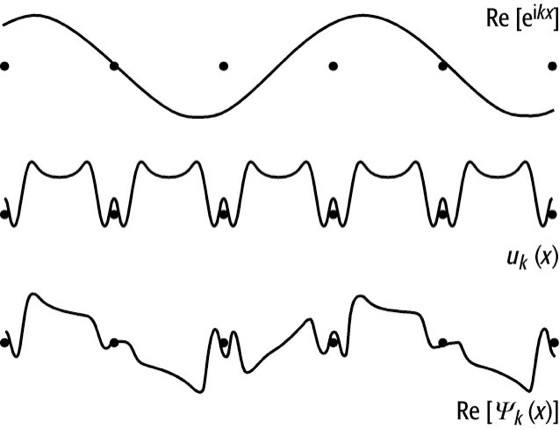Last edited:
Happiness
I figured out the problem. I'm going to write the resolution for the benefits of many others who have the same confusion as me and for my own future reference too.
They did not show how the theorem (5.49) or (4.198) could be applied to a linear combination of eigenfunctions. One eigenfunction one at a time, yes. But otherwise, no. I don't see how. And I made clear where my confusion is: ##\lambda_1\psi_1+\lambda_2\psi_2\neq k(\psi_1+\psi_2)##, for any constant ##k##.

This is the reason for confusion. Suppose ##\psi_1## and ##\psi_2## are separately a solution to the Schrondinger equation [5.48]. Then any linear combination ##\psi=c_1\psi_1+c_2\psi_2## will also be a solution to the Schrondinger equation [5.48].

Then by Bloch's theorem, ##\psi=c_1\psi_1+c_2\psi_2## will also satisfy [5.49]: $$\psi(x+a)=e^{iKa}\psi(x)$$ But in general, ##\psi_1## and ##\psi_2## may have different ##K##. In other words, ##\psi_1(x+a)=e^{iK_1a}\psi_1## and ##\psi_2(x+a)=e^{iK_2a}\psi_2##, but ##K_1\neq K_2## (mod ##\frac{2\pi}{a}##). Then, there won't be a common factor to factorise. Instead, we will end up with $$\psi(x+a)=e^{iK_1a}c_1\psi_1(x)+e^{iK_2a}c_2\psi_2(x)\neq e^{iKa}\psi(x)$$
As a result, [5.49] cannot be satisfied, contradicting Bloch's theorem.

So it seems that Bloch's theorem only works for ##\psi_1## alone and ##\psi_2## alone but not a linear combination of them. This has been the main message in all my replies, so far.

And it turns out I was right in this all along. Bloch's theorem indeed doesn't work for any arbitrary linear combination: it works for some linear combinations but not for all linear combinations.

You may revisit Griffiths' words again: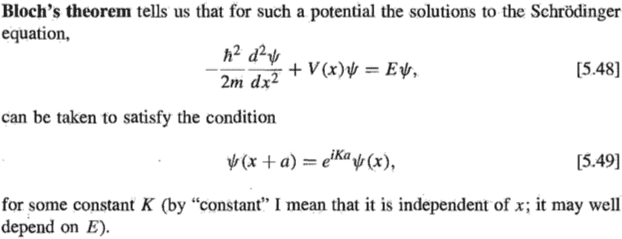You could blame it on his phrasing. What Bloch's theorem is saying is that there exist some solutions to the Schrondinger equation [5.48] that satisfy the condition [5.49]. It is not saying all solutions to the Schrondinger equation [5.48] will satisfy the condition [5.49].

I have spent a lot of time thinking about the resolution to this problem, only to realize that I had the wrong interpretation of Bloch's theorem. I wish Griffiths could have phrased this better, like how the mathematicians do it clearly, with key words like "for every", "there exists one", etc. This would have communicated the right idea across to the readers the first time, and avoid ambiguities and confusion and wasting time trying to figure out what went wrong.

It's also important to introduce the notations ##\psi_{\lambda_1}## and ##\psi_{\lambda_2}##, and differentiate them with ##\psi_1## and ##\psi_2##, because usually ##\psi_1## and ##\psi_2## are reserved to denote orthonormal eigenfunctions of H. One question you may be pondering is "why do B&J express ##\psi## only as a sum of 2 linearly independent solutions (4.186), and why not as a sum of 3 or more terms?" Certainly, H generally have more than 2 orthonormal eigenfunctions. So why stop at 2?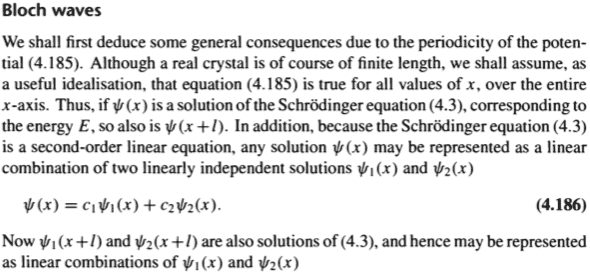It's because we are solving the Schrodinger equation at a certain value of E. And for a fixed value of E, the solution space is spanned by at most two linearly independent functions, since the Schrodinger equation is a second-order differential equation and would hence introduce two constants to the general solution. These two constants can be determined from ##\psi(x, 0)## and ##\frac{\partial\psi}{\partial t}(x, 0)##, giving us the solution to a particular scenario.

But why must we fix the value of E? It's because the K in Bloch's condition [5.49] may depend on E. So to make K truly constant, we should fix the value of E. (Of course, one needs to spend some time thinking over it to understand it for himself.)

Going back to the notations ##\psi_{\lambda_1}## and ##\psi_{\lambda_2}##. They are the eigenfunctions of D, with eigenvalues ##\lambda_1## and ##\lambda_2## respectively.

When you look at Griffiths' proof: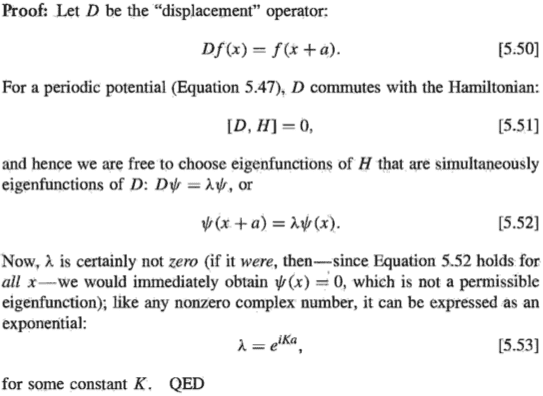You may think that since D and H commute, an eigenfunction of H, such as ##\psi_1##, is also an eigenfunction of D. But this is not true. Commutativity only tells us that there exist functions that are eigenfunctions of both D and H. So if ##\psi_1## are ##\psi_2## linearly independent eigenfunctions of the same energy, then ##\psi_{\lambda_1}=c_1\psi_1+c_2\psi_2##, for some ##c_1## and ##c_2##. But in general, ##\psi_{\lambda_1}\neq\psi_1##. Similarly, ##\psi_{\lambda_2}=c_1'\psi_1+c_2'\psi_2##, for some ##c_1'## and ##c_2'##.

Take a look at the example following Griffiths' proof: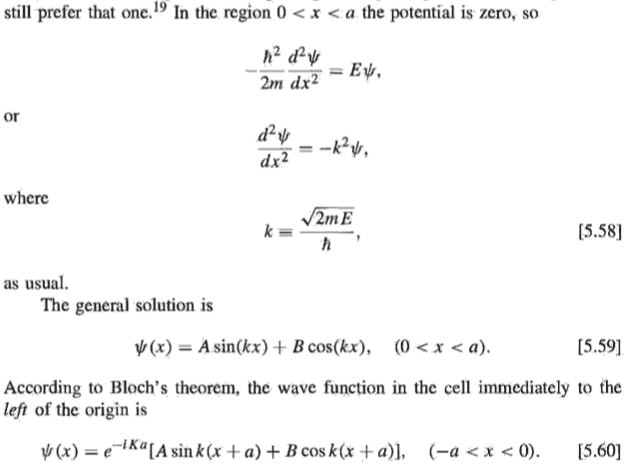Do you see why in [5.60] there is a common factor ##e^{-iKa}## even though I just said Bloch's theorem only works for one eigenfunction one at a time? In other words, could you explain why ##\sin k(x+a)## and ##\cos k(x+a)## have the same factor ##e^{-iKa}##? Why not ##e^{-iK_1a}## for ##\sin k(x+a)## and ##e^{-iK_2a}## for ##\cos k(x+a)##? How do we tell in what situation we should use the same ##K## and in what situation we should use ##K_1## and ##K_2##, like B&J's (4.196) below?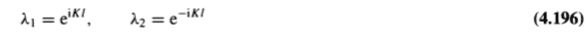The key is to understand that we apply Bloch's theorem to an eigenfunction of D, not of H. In other words, ##\psi(x+a)=e^{iKa}\psi_{\lambda_1}## but ##\psi(x+a)\neq e^{iKa}\psi_1##. In the above example, ##\sin(kx)## and ##\cos(kx)## are eigenfunctions of H, ie., ##\psi_1=\sin(kx)## and ##\psi_2=\cos(kx)##. But since ##\sin(kx)## and ##\cos(kx)## are eigenfunctions of the same energy, ##A\sin(kx)+B\cos(kx)## is also an eigenfunction of the same energy. Then, since D and H commute, out of all the eigenfunctions of H, one of them must also be an eigenfunction of D. In other words, there exist some values of ##A## and ##B## such that ##A\sin(kx)+B\cos(kx)=\psi_{\lambda_1}##. And this is [5.59] (see above), ##\psi(x)## being an eigenfunction of D, and ##A## and ##B## being certain values, not any arbitrary values. This is quite subtle, and requires some careful thought. [5.59] is presented by the book as the general solution. But when it is used with Bloch's theorem to form [5.60], we are searching from the pool of the general solution, a particular solution that is an eigenfunction of D, with A and B taking on certain values. Hence, after displacing to the left by ##a##, we have ##\psi_{\lambda_1}(x-a)=e^{-iK_1a}\psi_{\lambda_1}(x)=e^{-iK_1a}[A\sin(kx)+B\cos(kx)]##, which is [5.60] after substituting ##x## by ##(x+a)##.

Last edited:
You are still missing the point. The point lies in introducing the periodic Born-von Karman boundary conditions (the steps [5.55] and [5.56] you left out). While one assumes a symmetry for a shift by one single lattice constant a only, the value of K is already defined perfectly by the reciprocal lattice vector G and there is just this one discrete value of K, which works for all states.

However as you apply periodic boundary conditions, you also enforce shifts by integer multiples N of a to be a symmetry operation. Here, you get more allowed values for K. Every fraction $\frac{nG}{N}$ will now also form an allowed K. Starting from this point, you need to worry about phase shifts of less than 2 pi per unit cell, which cause wave functions of different periodicity to appear. However, this starts only as you take displacements along several multiples of a into account.
You can then go on to find the energy of each of these states. For large N, this means that k becomes quasi-continuous as the states are too close to be able to resolve the discreteness in k. For systems consisting of few unit cells, you can see the discreteness in experiments.

Happiness
You are still missing the point. The point lies in introducing the periodic Born-von Karman boundary conditions
This wasn't the issue I had.

there is just this one discrete value of K
There are two values of K for each energy E.

which works for all states
Not quite clear what you mean. States that are eigenfunctions of D? States of the same energy?

However as you apply periodic boundary conditions, you also enforce shifts by integer multiples N of a to be a symmetry operation. Here, you get more allowed values for K. Every fraction ##\frac{nG}{N}## will now also form an allowed K. Starting from this point, you need to worry about phase shifts of less than 2 pi per unit cell, which cause wave functions of different periodicity to appear. However, this starts only as you take displacements along several multiples of a into account.
Ok, there may be other ways to get [5.56]. But the book's approach is much easier. It's just one line. And this wasn't my issue. The priority should be on the accurate identifying of the cause of the confusion and addressing it using the simplest tool, without too much unnecessary information. Nonetheless, thank you for your advice.

Mentor
What Bloch's theorem is saying is that there exist some solutions to the Schrondinger equation [5.48] that satisfy the condition [5.49]. It is not saying all solutions to the Schrondinger equation [5.48] will satisfy the condition [5.49].

No, Bloch's theorem does indeed say that all solutions of [5.48] given that the potential satisfies [5.47] satisfy the condition [5.49].

The priority should be on the accurate identifying of the cause of the confusion

What confusion? As far as I can see, the only confusion in this thread is that you keep making incorrect claims.

•Happiness
No, Bloch's theorem does indeed say that all solutions of [5.48] given that the potential satisfies [5.47] satisfy the condition [5.49].
Ok could you explain how do you know that it's impossible for a solution to Schrodinger equation with such a potential to not satisfy the condition [5.49]?

Consider this counter example. Let ##\psi_1## and ##\psi_2## be the solution with energy ##E_1## and ##E_2## to the Schrodinger equation with the Dirac comb potential respectively. Then, ##\psi=\psi_1+\psi_2## must be a solution too (if with the appropriate normalisation). But ##\psi## does not satisfy the condition [5.49], because ##\psi(x+a)=e^{iK_1a}\psi_1(x)+e^{iK_2a}\psi_2(x)## and in general, ##K_1\neq K_2## (mod ##\frac{2\pi}{a}##), since ##K_1## depends on ##E_1## and ##K_2## depends on ##E_2##.

Last edited:
Mentor
could you explain how do you know that it's impossible for a solution to Schrodinger equation with such a potential to not satisfy the condition [5.49]?

Let ##\psi_1## and ##\psi_2## be the solution with energy ##E_1## and ##E_2## to the Schrodinger equation with the Dirac comb potential respectively. Then,##\psi=\psi_1+\psi_2## must be a solution too.

...is incorrect. A linear combination of eigenstates of the Hamiltonian is not, in general, also an eigenstate. And Bloch's Theorem only applies to eigenstates of the Hamiltonian--more precisely, to states that are common eigenstates of both the Hamiltonian ##H## and the displacement operator ##D##.

Happiness
And Bloch's Theorem only applies to eigenstates of the Hamiltonian--more precisely, to states that are common eigenstates of both the Hamiltonian H and the displacement operator D.
No, Bloch's theorem does indeed say that all solutions of [5.48] given that the potential satisfies [5.47] satisfy the condition [5.49].

Mentor

No. Equation [5.48] is the eigenvalue equation for the Hamiltonian. Only eigenstates of the Hamiltonian satisfy it.

Happiness
No. Equation [5.48] is the eigenvalue equation for the Hamiltonian. Only eigenstates of the Hamiltonian satisfy it.
But there may exist eigenstates of the Hamiltonian that satisfy it (5.48 modified with 5.47) that are not eigenstates of D and so would not satisfy [5.49].

Mentor
there may exist eigenstates of the Hamiltonian that satisfy it (5.48 modified with 5.47) that are not eigenstates of D and so would not satisfy [5.49]

No, there can't. Since ##D## and ##H## commute, every state that is an eigenstate of one must be an eigenstate of the other. (More precisely, this is true if each operator has a complete set of non-degenerate eigenstates; degeneracy complicates things somewhat but is not an issue for this discussion.) Griffiths' proof makes explicit use of this theorem. It is a basic theorem of QM; are you not aware of it?

Last edited:
Happiness
No, there can't. Since ##D## and ##H## commute, every state that is an eigenstate of one must be an eigenstate of the other. (More precisely, this is true if each operator has a complete set of non-degenerate eigenstates; degeneracy complicates things somewhat but is not an issue for this discussion.) Griffiths' proof makes explicit use of this theorem. It is a basic theorem of QM; are you not aware of it?
I'm aware of it. Isn't degeneracy an issue here since we are talking about eigenstates of H with the same E? ##\sin(kx)## and ##\cos(kx)## have the same eigenvalue E. Doesn't this mean a degeneracy of 2?

Mentor
Isn't degeneracy an issue here since we are talking about eigenstates of H with the same E?

Degeneracy complicates the proof of the theorem somewhat, but it still applies.

In the specific case you give, ##\sin (kx)## and ##\cos (kx)## are both eigenstates of ##D## for appropriate values of ##k##, and only those values of ##k## will give eigenstates of ##H##.

Happiness
Degeneracy complicates the proof of the theorem somewhat, but it still applies.
In cases with degeneracy, there may exist eigenstates of H that are not eigenstates of D, so Griffith's proof does not prove Bloch's theorem for these cases.
In the specific case you give, ##\sin (kx)## and ##\cos (kx)## are both eigenstates of ##D## for appropriate values of ##k##, and only those values of ##k## will give eigenstates of ##H##.
k can only take on certain values. This is correct. These values corresponds to the allowed energy values. For a certain allowed energy value, ##\sin (kx)##, ##\cos (kx)##, ##2\sin (kx)+\cos (kx)## and in fact all other linear combinations are all eigenstates of H. But only 2 of them (at the most) are eigenstates of D. They are the eigenvectors of the matrix in (4.189).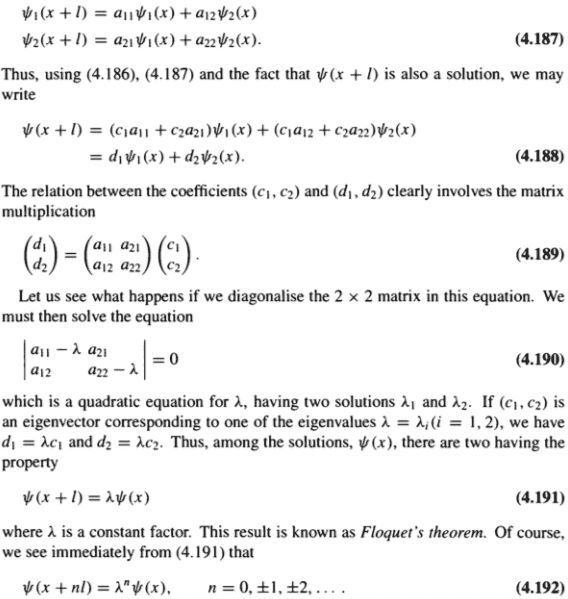Reference: QM 2nd ed., B&J, p183

Since the other eigenstates of H are not eigenstates of D, the following sentence must be false.
No, Bloch's theorem does indeed say that all solutions of [5.48] given that the potential satisfies [5.47] satisfy the condition [5.49].

Last edited:
Mentor
For a certain allowed energy value, ##\sin (kx)##, ##\cos (kx)##, ##2\sin (kx)+\cos (kx)## and in fact all other linear combinations are all eigenstates of H. But only 2 of them (at the most) are eigenstates of D.

You are ignoring the fact that the value of ##k## cannot be chosen arbitrarily; it must satisfy ##k = n \pi / a##, where ##n## is an integer and ##a## is the "spacing" in the potential ##V(x)##. Otherwise we won't have an eigenstate of ##H## (or ##D## for that matter). And for any given energy eigenvalue, all eigenfunctions must have the same ##k##.

For any such value of ##k##, we have ##\sin k (x + a) = \sin (kx + n \pi) = \sin (kx)##, and similarly for ##\cos k(x + a)##. Then any function ##\psi (x) = a \sin (kx) + b \cos (kx)## will give

$$\psi(x + a) = a \sin k (x + a) + b \cos k(x + a) = a \sin(kx + n \pi) + b \cos(kx + n \pi) \\ = a \sin (kx) + b \cos (kx) = \psi(x)$$

I think the source you reference is considering the more general case where ##\psi_1## and ##\psi_2## do not have the same energy eigenvalue and hence do not have the same ##k##.

In cases with degeneracy, there may exist eigenstates of H that are not eigenstates of D, so Griffith's proof does not prove Bloch's theorem for these cases.

See above.

Happiness
it must satisfy k = ##\pi##/a, where a is the "spacing" in the potential V(x)
Why? I know ##K=\frac{2\pi n}{Na}## cannot be arbitrarily chosen. For k, could you explain further? Is this only for this specific example?
I think the source you reference is considering the more general case where ##\psi_1## and ##\psi_2## do not have the same energy eigenvalue and hence do not have the same ##k##.
They have the same energy: "corresponding to the energy E" (line 4).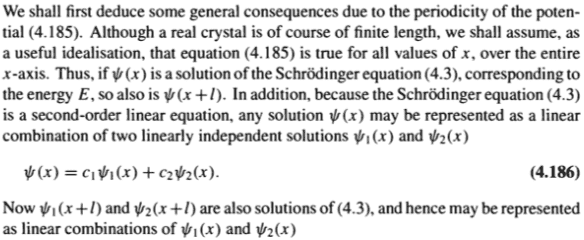Last edited:
Mentor
Why?

Because it has to be an eigenstate of ##D##: any eigenfunction ##\psi(x)## of ##D## must be periodic with period ##a##. I misspoke in my earlier post; I should have said "it won't be an eigenstate of ##D##" instead of "it won't be an eigenstate of ##H##".

Mentor
Is this only for this specific example?

The logic I gave should work for any functions that are periodic with the same periodicity (which in this case will need to be ##a## divided by an integer).

Mentor
I misspoke in my earlier post; I should have said "it won't be an eigenstate of ##D##" instead of "it won't be an eigenstate of ##H##".

Perhaps it will help to rephrase the argument I was making as follows:

Given any value of ##k## (not assuming any restriction on ##k## at the start of the argument), the functions ##\sin(kx)## and ##\cos(kx)## form a basis of a space of functions that all have the same periodicity. Assume that these functions are all eigenstates of ##H## (if anyone of them is, they all are because they all have the same ##k##, and if none of them are, we don't care about this value of ##k## anyway). In order for the conditions needed for Bloch's Theorem to apply at all, at least one of the functions must also be an eigenfunction of ##D##. And if anyone of them is, they all are, since they all have the same periodicity, and periodicity is the only criterion that determines whether a function is an eigenfunction of ##D##. And the condition that at least one of the functions must be an eigenfunction of ##D## is what places the restriction on ##k##; only certain values of ##k## allow any of the functions to be an eigenfunction of ##D##.